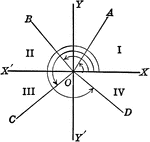Axes showing trigonometric angles, and quadrants.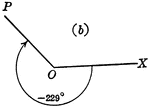### -229 Degree Trigonometric Angle

Trigonometric angle -229 degrees.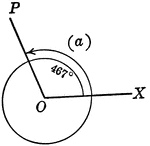### 467 Degree Trigonometric Angle

Trigonometric angle 467 degrees.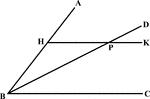### Angle Bisector Drawn Parallel to A Side

Illustration to show that if through any point in the bisector of an angle a line is drawn parallel…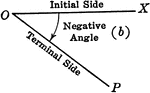### Negative Trigonometric Angle

Negative trigonometric angle with initial and terminal sides labeled.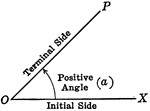### Positive Trigonometric Angle

Positive trigonometric angle with initial and terminal sides labeled.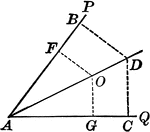### Angle Bisector

Illustration showing that the bisector of a given angle is the locus of points equidistant from the…### Perpendicular Bisector of a Line

Illustration showing that the perpendicular bisector of a given line is the locus of points equidistant…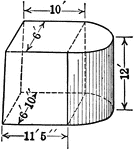### Composite Figure of Quadrilateral Frustum With Half of a Cylinder Attached

An illustration of a composite figure made up of a quadrilateral frustum and half of a cylinder. Frustum…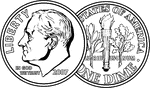### Both sides of a Dime

An illustration of both sides of a U.S. Dime.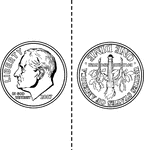### Two Sided Dime

An illustration of both sides of a U.S. Dime that can be cutout and folded.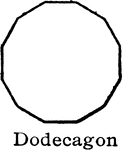### Dodecagon

"A polygon of...twelve sides is [a] dodecagon." —Hallock 1905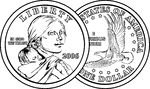### Both sides of a Dollar

An illustration of both sides of a U.S. Dollar Coin.### Two Sided Dollar

An illustration of both sides of a U.S. Dollar Coin that can be cutout and folded.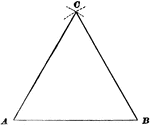### Construction Of Equilateral Triangle

Illustration used to show how to draw an equilateral triangle when given one side.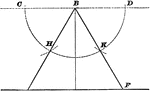### Construction Of Equilateral Triangle

Illustration used to show how to draw an equilateral triangle when given the altitude.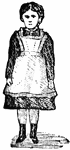### Girl Standing

A young girl standing with her arms at her sides.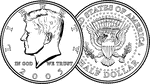### Both sides of a Half Dollar

An illustration of both sides of a U.S. Half Dollar.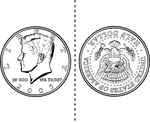### Two Sided Half Dollar

An illustration of both sides of a U.S. Half Dollar that can be cutout and folded.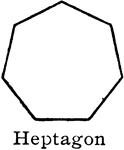### Heptagon

"A polygon of...seven sides [is] a heptagon." —Hallock 1905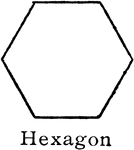### Hexagon

"A polygon of...six sides a [is] a hexagon." —Hallock 1905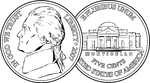### Both sides of a Nickel

An illustration of both sides of a U.S. nickel.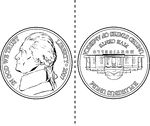### Two Sided Nickel

An illustration of both sides of a U.S. nickel that can be cutout and folded.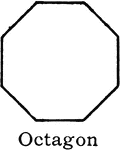### Octagon

"A polygon of...eight sides [is] an octagon." —Hallock 1905### Octahedron

An octahedron, or double four-sided pyramid. It has 8 sides.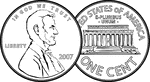### Both sides of a Penny

An illustration of both sides of a U.S. Penny.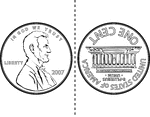### Two Sided Penny

An illustration of both sides of a U.S. Penny that can be cutout and folded.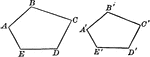### Similar Polygons (Pentagons) That Can Be Used To Show Proportionality

Illustration that shows similar polygons (pentagons) that can be used to show proportionality.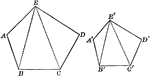### Similar Polygons (Pentagons) That Can Be Used To Show Proportionality

Illustration that shows similar polygons (pentagons) that can be used to show proportionality.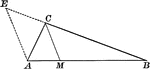### Angle Bisector of Triangle Dividing Opposite Side Into Proportionate Segments

Illustration to show that an angle bisector of a triangle divides the opposite side into segments which…### Exterior Angle Bisector of Triangle Dividing Opposite Side Into Proportionate Segments

Illustration to show that a bisector of a an exterior angle of a triangle divides the opposite side…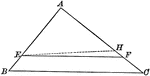### Triangle Divided by Parallel Lines to Form Proportionate Triangles

Illustration to show that if a line divides two sides of a triangle proportionally, it is parallel to…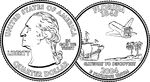### Both sides of a Quarter

An illustration of both sides of a U.S. Quarter.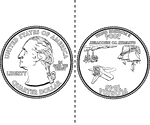### Two Sided Quarter

An illustration of both sides of a U.S. Quarter that can be cutout and folded.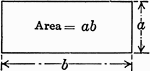### Rectangle with Lengths a and b with Area ab

An illustration of a rectangle with lengths a and b and area ab.### Rectangle with Lengths a, b, and c with Areas ac and bc

An illustration of composite rectangle with lengths a, b, and c with area ac + bc. This illustration…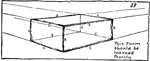### Rectangular Prism

A rectangular prism with labeled sides: AAAA are the four vertical parallel corners. BBBB and CCCC are…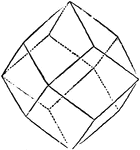### Rhombic Dodecahedron

A geometric shape containing twelve faces.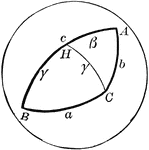### Side Comparison of Spherical Triangle

Diagram used to prove the theorem: "In a spherical triangle, the greater side is opposite the greater…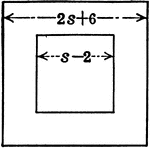### Hollow Square with Dimensions of 2s+6 and s-2

An illustration of a hollow square (square within square) with outer square having a side of 2s+6 and…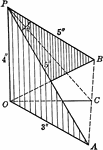### Two Squares With Sides of Lengths 3,4,5 Placed at Right Angles to Each Other

Two set squares, whose sides are 3,4, and 5 in., are placed so that their 4-in. sides and right angles…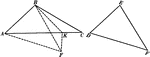### 2 Triangles Theorem

Illustration used to prove that "If two triangles have two sides of one equal respectively to two sides…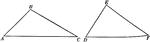### 2 Triangles Theorem

Illustration used to prove that "If two triangles have two sides of one equal respectively to two sides…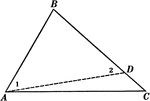### Sides of Triangle Theorem

Illustration used to prove that "If two sides of a triangle are unequal, the angle opposite the greater…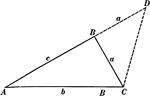### Sides of Triangle Theorem

Illustration used to prove that "The sum of any two sides of a triangle is greater than the third side."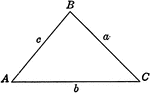### Triangle

Triangle with sides a, b, c and angles A, B, C labeled.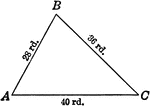### Triangle 28, 36, 40

Triangle with sides labeled with numerals 28, 36, 40 and angles A, B, C labeled.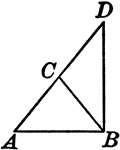### Leg Produced through the Vertex of an Isosceles Triangle

Illustration to show that if one of the legs of an isosceles triangle is produced through the vertex…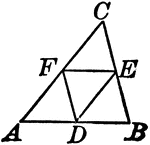### Midpoints of Triangle Divide Triangle into Four Equal Triangles

Illustration to show that if the lines joining the middle points of the sides of a triangle divide the…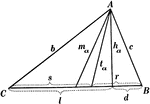### Parts Of A Triangle

Illustration used to show the various parts of a triangle: sides, angles, medians, altitudes, bisectors,…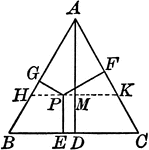### Perpendiculars Dropped Any Point in the Equilateral Triangle

Illustration to show the perpendiculars dropped from any point in the equilateral triangle to the three…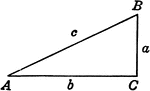### Right Triangle ABC

Right triangle with sides a, b, c and angles A, B, C labeled.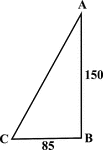### Right Triangle With Sides 85 and 150

Illustration of a right triangle with legs labeled 150 and 85. This could be used to find the hypotenuse…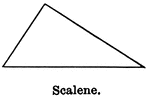### Scalene Triangle

Illustration showing a scalene triangle (one that has no two sides equal).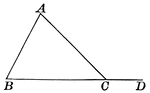### Triangle With Segment Extended for Exterior Angle

Illustration showing a triangle with an exterior segment drawn to show and exterior angle. This can…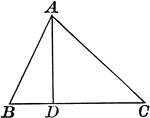### Triangle With Interior Segment

Illustration showing a triangle with an interior segment drawn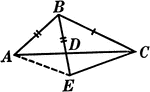### Segments Labeled In A Triangle

Illustration of triangle ABC with BE extended through the triangle at point D. Segment AB is equal to…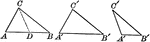### Proof of Equal Triangles Drawing

Illustration to show if two triangles have two sides of the one equal, respectively, to two sides of…